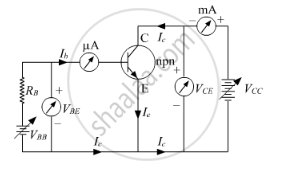HSC Science (Electronics) 12th Board ExamMaharashtra State Board
Share

# Draw a Circuit Diagram for Studying the Input and Output Characteristics of a N-p-n Transistor in Common Emitter Configuration. Using the Circuit, Explain How Input, Output Characteristics Are Obtained - HSC Science (Electronics) 12th Board Exam - Physics

ConceptJunction Transistor Transistor as an Amplifier (Ce-configuration)

#### Question

Draw a circuit diagram for studying the input and output characteristics of a n-p-n transistor in common emitter configuration. Using the circuit, explain how input, output characteristics are obtained

#### SolutionUsing the circuit, input characteristics are represented by a graph showing variation between the base current and the base-emitter voltage. This characteristic or graph is further used to evaluate input dynamic resistance of the transistor as the slope of the graph. Similarly, the same circuit is used to find the output characteristics of the circuit using the graph representing the variation of the collector current and the emitter voltage, keeping base current fixed. Here, the output dynamic resistance is evaluated as the slope of the output characteristics graph.

Is there an error in this question or solution?

#### APPEARS IN

2016-2017 (July) (with solutions)
Question 6.1 | 3.00 marks

#### Video TutorialsVIEW ALL 

Solution Draw a Circuit Diagram for Studying the Input and Output Characteristics of a N-p-n Transistor in Common Emitter Configuration. Using the Circuit, Explain How Input, Output Characteristics Are Obtained Concept: Junction Transistor - Transistor as an Amplifier (Ce-configuration).
S